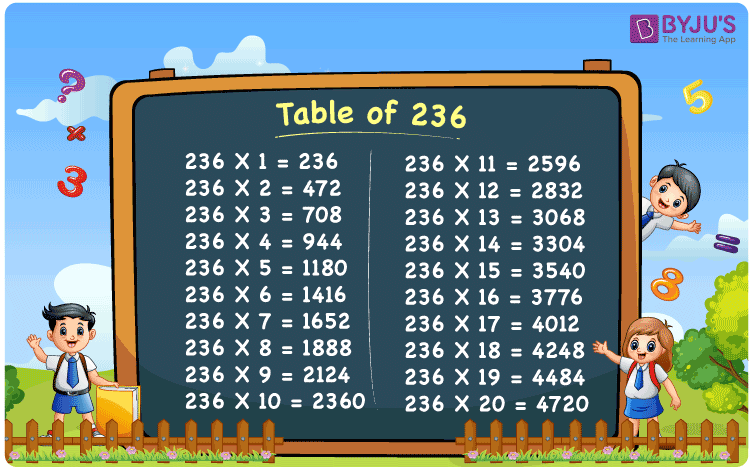Checkout JEE MAINS 2022 Question Paper Analysis : Checkout JEE MAINS 2022 Question Paper Analysis :

# Table of 236

The table of 236 is the 236 times table in Maths, which shows the results as the multiples of 236. Memorizing the 236 times table helps the students and the candidates who are preparing for competitive examinations, as it saves time while solving the problems. Here, we have provided the table of 236 in the tabular format and PDF for the multiplication table of 236, which can be downloaded by clicking on the link below.

## Table of 236 Chart## What is the 236 Times Table?

The 236 times table uses the repeated addition and the multiplication process, which is shown below.

For example, 236 × 5 = 1180.

It is also written as 236 + 236 + 236 + 236 + 236 =1180.

 236 × 1 = 236 236 236 × 2 = 472 236 + 236 = 472 236 × 3 = 708 236 + 236 + 236 = 708 236 × 4 = 944 236 + 236 + 236 + 236 = 944 236 × 5 = 1180 236 + 236 + 236 + 236 + 236 = 1180 236 × 6 = 1416 236 + 236 + 236 + 236 + 236 + 236 = 1416 236 × 7 = 1652 236 + 236 + 236 + 236 + 236 + 236 + 236 = 1652 236 × 8 = 1888 236 + 236 + 236 + 236 + 236 + 236 + 236 + 236 = 1888 236 × 9 = 2124 236 + 236 + 236 + 236 + 236 + 236 + 236 + 236 + 236 = 2124 236 × 10 = 2360 236 + 236 + 236 + 236 + 236 + 236 + 236 + 236 + 236 + 236 = 2360

## Multiplication Table of 236

The below table shows the multiplication table of 236, which gives the list of the first 20 multiples of 236.

 236 × 1 = 236 236 × 2 = 472 236 × 3 = 708 236 × 4 = 944 236 × 5 = 1180 236 × 6 = 1416 236 × 7 = 1652 236 × 8 = 1888 236 × 9 = 2124 236 × 10 = 2360 236 × 11 = 2596 236 × 12 = 2832 236 × 13 = 3068 236 × 14 = 3304 236 × 15 = 3540 236 × 16 = 3776 236 × 17 = 4012 236 × 18 = 4248 236 × 19 = 4484 236 × 20 = 4720

## Solved Examples on the Table of 236

Example 1:

What is the 20th multiple of 236?

Solution:

Using the multiplication table of 236,

The 20th multiple of 236 is 4720.

I.e., 20 × 236 = 4720.

Example 2:

If Arjun is asked to write 8 poems in a day, then how many poems will he write in 236 days?

Solution:

In a day, Arjun writes 8 poems.

Then, the number of poems he will write in 236 days = 8 × 236

= 1888

Therefore, the number of poems he will write in 236 days is 1888 poems.

## Frequently Asked Questions on the Table of 236

### What is the table of 236?

The table of 236 shows the list of multiples of 236. The 236 times can be obtained using both the addition and multiplication process.

### Write 236 times tables up to 10 times.

236 × 1 = 236, 236 × 2 = 472, 236 × 3 = 708, 236 × 4 = 944, 236 × 5 = 1180, 236 × 6 = 1416, 236 × 7 = 1652, 236 × 8 = 1888, 236 × 9 = 2124, 236 × 10 = 2360.

### What is 236 times 17?

236 times 17 is 4012. I.e., 236 × 17 = 4012.

### How many times 236 is 2124?

9 times 236 is 2124. I.e., 236 × 9 = 2124.